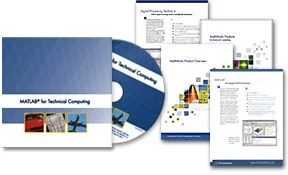# Get Free Interactive MATLAB CD and Technical Kit

Posted byIf you want to work on some really complex math computations such as?

• Signal and image processing
• Financial modeling and analysis
• Algorithm development
• Data visualization
• Data analysis
• Numeric computation

Then MATLAB may be the perfect solution for you,I have been using Matlab for my Project and lab works  for months  but I was not aware of many of things in the software. If you are a beginner  or if you are also someone who is looking for the depth MatLab, then I recommend you to avail this free offer.

No one likes to read books which are complex and which is not that easy to understand. There are some tutorials, but they are not that informative and not complete also. That is why the best thing that you can get is a matlab free tutorial CD.

Software developers MathWorks.in are offering an interactive CD loaded with product demos and webinars, plus a technical kit that contains data sheets for MATLAB and Signal Processing Toolbox, the Technical Computing brochure, and the MathWorks Product Overview.

In this CD you will find 11 important lecture. To see the lecture u
have to use flash player
This CD includes Following:

1. Introduction to MATLAB

2. Data Analysis with MATLAB for Excel® Users

3. Algorithm Development with MATLAB for C/C++ Products

4. Introduction to Statistics with MATLAB Products

5. Introduction to Optimization with MATLAB Products

6. Application Deployment with MATLAB

7. MATLAB for Signal Processing

8. Image Processing Using MATLAB

9. Data Acquisition with MATLAB Products

10. Using MATLAB to Develop Financial Products

11. Introduction to MATLAB for Life Scientists